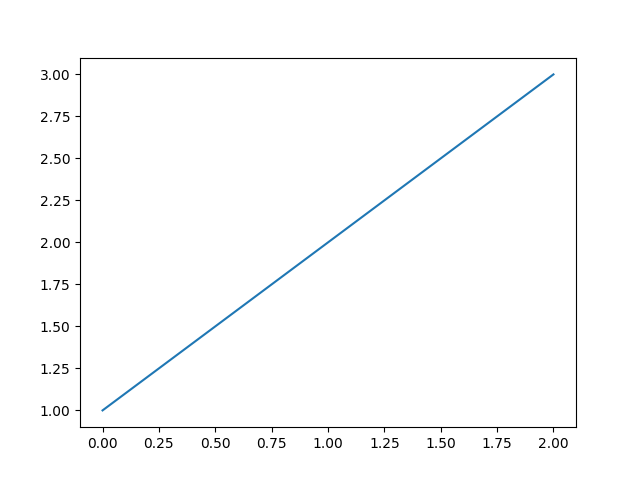# Capturing output representations#

This example demonstrates how the configuration `capture_repr` (Controlling what output is captured) works. The default `capture_repr` setting is `capture_repr: ('_repr_html_', '__repr__')` and was used when building the Sphinx-Gallery documentation. The output that is captured with this setting is demonstrated in this example. Differences in outputs that would be captured with other `capture_repr` settings is also explained.

Nothing is captured for the code block below because no data is directed to standard output and the last statement is an assignment, not an expression.

```# example 1
a = 2
b = 10
```

If you did wish to capture the value of `b`, you would need to use:

```# example 2
a = 2
b = 10
b  # this is an expression
```
```10
```

Sphinx-Gallery first attempts to capture the `_repr_html_` of `b` as this is the first ‘representation’ method in the `capture_repr` tuple. As this method does not exist for `b`, Sphinx-Gallery moves on and tries to capture the `__repr__` method, which is second in the tuple. This does exist for `b` so it is captured and the output is seen above.

A pandas dataframe is used in the code block below to provide an example of an expression with a `_repr_html_` method.

```# example 3
import pandas as pd

df = pd.DataFrame(data={"col1": [1, 2], "col2": [3, 4]})
df
```
col1 col2
0 1 3
1 2 4

The pandas dataframe `df` has both a `__repr__` and `_repr_html_` method. As `_repr_html_` appears first in the `capture_repr` tuple, the `_repr_html_` is captured in preference to `__repr__`.

For the example below, there is data directed to standard output and the last statement is an expression.

```# example 4
print("Hello world")
a + b
```
```Hello world

12
```

Statsmodels tables should also be styled appropriately:

```# example 5
import numpy as np
import statsmodels.iolib.table

statsmodels.iolib.table.SimpleTable(np.zeros((3, 3)))
```
 0 0 0 0 0 0 0 0 0

`print()` outputs to standard output, which is always captured. The string `'Hello world'` is thus captured. A ‘representation’ of the last expression is also captured. Again, since this expression `a + b` does not have a `_repr_html_` method, the `__repr__` method is captured.

## Matplotlib output#

Matplotlib function calls generally return a Matplotlib object as well as outputting the figure. For code blocks where the last statement is a Matplotlib expression, a ‘representation’ of the object will be captured, as well as the plot. This is because Matplotlib objects have a `__repr__` method and our `capture_repr` tuple contains `__repr__`. Note that Matplotlib objects also have a `__str__` method.

In the example below, `matplotlib.pyplot.plot()` returns a list of `Line2D` objects representing the plotted data and the `__repr__` of the list is captured as well as the figure:

```import matplotlib.pyplot as plt

plt.plot([1, 2, 3])
``````[<matplotlib.lines.Line2D object at 0x7fca8e244820>]
```

To avoid capturing the text representation, you can assign the last Matplotlib expression to a temporary variable:

```_ = plt.plot([1, 2, 3])
```Alternatively, you can add `plt.show()`, which does not return anything, to the end of the code block:

```plt.plot([1, 2, 3])
plt.show()
```## The `capture_repr` configuration#

The `capture_repr` configuration is `('_repr_html_', '__repr__')` by default. This directs Sphinx-Gallery to capture ‘representations’ of the last statement of a code block, if it is an expression. Sphinx-Gallery does this according to the order ‘representations’ appear in the tuple. With the default `capture_repr` setting, `_repr_html_` is attempted to be captured first. If this method does not exist, the `__repr__` method would be captured. If the `__repr__` also does not exist (unlikely for non-user defined objects), nothing would be captured. For example, if the the configuration was set to `'capture_repr': ('_repr_html_')` nothing would be captured for example 2 as `b` does not have a `_repr_html_`. You can change the ‘representations’ in the `capture_repr` tuple to finely tune what is captured in your example `.py` files.

To only capture data directed to standard output you can set `capture_repr` to be an empty tuple: `capture_repr: ()`. With this setting, only data directed to standard output is captured. For the examples above, output would only be captured for example 4. Although the last statement is an expression for examples 2, 3 and 4 no ‘representation’ of the last expression would be output. You would need to add `print()` to the last expression to capture a ‘representation’ of it. The empty tuple setting imitates the behaviour of Sphinx-Gallery prior to v0.5.0, when this configuration was introduced.

Total running time of the script: (0 minutes 2.972 seconds)

Estimated memory usage: 20 MB

Gallery generated by Sphinx-Gallery